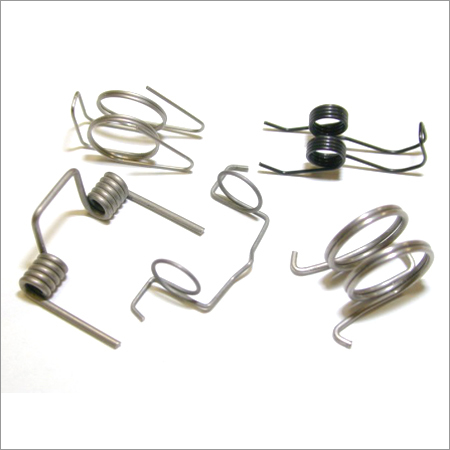0

# Torsion SpringTorsion Spring
Price And Quantity
• 10
• Unit/Units
• 10.00-
• 10INR
Product Description

Helical springs used to apply torque or store rotational energy are commonly referred to as torsion or double torsion. Torque by definition is a force that produces rotation. A torsion spring exerts a force (torque) in a circular arc and the arms rotate about the central axis. The stress is in bending and not in torsion. It is customary to specify torque with deflection or with the arms at a definite position. Formulas for torque are in pound-inches or inches-pounds. If ounce-inches are specified the value should be divided by 16 to use the formulas in the inch-pound system

When a force is specified at a distance from a centerline or the torque, the distance multiplies and is called momentum, which is equal to the force. Force can be in pounds or ounces with the distance in inches or the force can be in meters with the distance in millimeters. Formulae for torque are based on the tangent to the arc of rotation with a rod to support the spring. The stress in bending caused by the moment is identical in magnitude to the torque, provided that a rod is used.

Torsion and double torsion, whose ends are rotated in angular deflection, offer resistance to externally applied torque. The wire itself is subjected to bending stresses rather than torsional stresses, as might be expected from the name. Torsion springs of this type are usually closely wound, reduced in coil diameter and increase in body length as they are deflected. The designer must consider the effects of friction and arm deflection on the torque.

Special types of torsion springs include a double torsion spring having a space between the coils to minimize friction. Double torsion springs consist of one right-hand and one left-hand coil section connected together and working in parallels. The sections are designed separately with the total torque exerted being the sum of the two, thus called a double torsion.

Features: